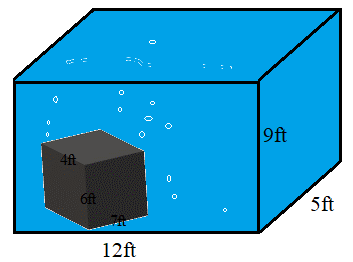# Volume

The volume of an 3D object is the total quantity of space the shape occupies. The volume of an object is measured in cubic units such as cubic centimeters, cubic inch, cubic foot, cubic meter, etc.. Finding the volume of an object can help us to determine the amount required to fill that object like the amount of water needed to fill a bottle, an aquarium or a water tank.

## Volume of Common Shapes

### Cube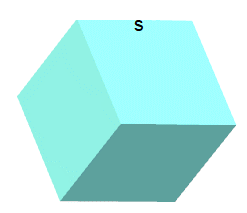The volume of a cube with length s is calculated by:

$$V = s^3$$

### Cuboid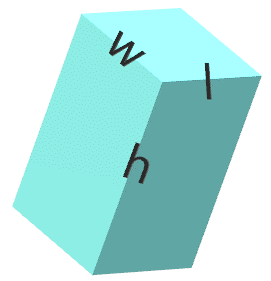The volume of a cuboid (rectangular prism) with length $$l$$, width $$w$$ and height $$h$$ is calculated by:

$$V = l w h$$

### Cone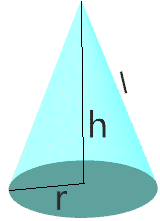The volume of a cone with height $$h$$, slant $$l$$ and radius $$r$$ is calculated by:

$$V = \cfrac{1}{3} \pi r^2 h$$

### Cylinder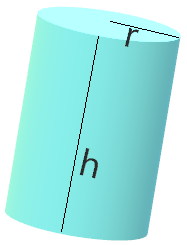The volume of a cylinder with radius $$r$$ and height $$h$$ is calculated by:

$$V = \pi r^2 h$$

### Triangular Prism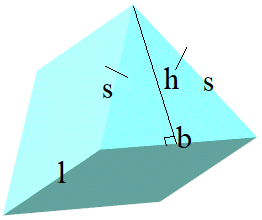The volume of a triangular prism is calculated by:

$$V = \cfrac{1}{2} b h l$$

### Sphere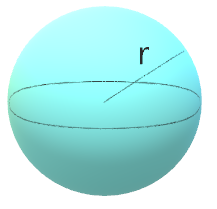The volume of a sphere is calculated as:

$$V = \cfrac{4}{3} \pi r^3$$

Note that the volume of shapes like prisms and cylinders can be calculated as:

$$V = A_{base}h$$

For example if the area of the base of a pentagonal prism is $$12 \; [\text{cm}^2]$$ and the height is $$20 \; [\text{cm}]$$, the volume is:

$$V = A_{base}h$$

$$V = (12)(20)$$

$$V = 240 \; [\text{cm}^3]$$

A cubic shaped box is $$50\;[cm]$$ by $$50 \;[cm]$$ by $$50 \;[cm]$$. A shipping crate is packed with $$18$$ of these boxes, with no extra space in the crate. What is the volume of the crate in meters?

Find the volume of a can of soda. The radius of the base is $$4\;[cm]$$ and the height is $$13\;[cm]$$.

A water tank is $$12\; \text{feet}$$ long, $$5\; \text{feet}$$ wide, and $$9\; \text{feet}$$ high. A solid metal box which is $$7\; \text{feet}$$ long, $$4\; \text{feet}$$ wide, and $$6\; \text{feet}$$ high is sitting at the bottom of the tank. The tank is filled with water. What is the volume of the water in the tank?